# Predicting Housing Prices Using Linear Regression¶

### 1.1.0 Import Packages¶

In :
import numpy as np
import matplotlib.pyplot as plt
import pandas as pd
import seaborn as sns
from sklearn.metrics import explained_variance_score,r2_score
from sklearn import linear_model


### 2.1.0 Pre - Processing Raw Data¶

• Features Dropped - due to irrelevance
• SALE TYPE,SOLD DATA,FAVORITE,INTERESTED,STATE,STATUS,NEXT OPEN HOUSE START TIME,NEXT OPEN HOUSE END TIME,SOURCE
• Deleted 11 data points [22,32] - as they were housing plans and not real houses
• HOA/MONTH - ignoring this feature as it has too many 60 missing data points to be reliable
In :
# raw data
raw_df.shape

Out:
(131, 27)
In :
# modified data
df.shape

Out:
(120, 18)

### 2.2.0 Measures of Central Tendencies¶

In :
# some state on the numerical features to get a general sense of the dataset

cols = ['PRICE','BEDS','BATHS','SQUARE FEET','$/SQUARE FEET','HOA/MONTH','DAYS ON MARKET'] mean = {} std = {} median = {} dfprint = pd.DataFrame(index=cols) for col in cols: std[col] = df[col].std() mean[col] = df[col].mean() median[col] = df[col].median() dfprint['MEAN'] = mean.values() dfprint['MEDIAN'] = median.values() dfprint['STD DEVIATION'] = std.values() dfprint.head(len(cols))  Out: MEAN MEDIAN STD DEVIATION PRICE 775613.366667 779895.0 355431.998217 BEDS 3.500000 4.0 1.466718 BATHS 2.638393 2.5 0.619539 SQUARE FEET 2366.447368 2404.5 881.377202$/SQUARE FEET 317.517544 321.5 101.471757
HOA/MONTH 141.200000 109.0 101.684757
DAYS ON MARKET 69.458333 18.0 112.333380

### 2.3.0 Determining the viability of the features¶

• Chosen Features as input exploration
• BEDS
• BATHS
• SQUARE FEET
• LOT SIZE
• Original Feature space Suggested Feature space
27 4
• A useful indicator might be the correlation matrix between the chosen features.

• Covariance between the 2 random variables $Cov(\vec{X},\vec{Y})$ is calculated as below
• $Cov(\vec{X},\vec{Y}) = \dfrac{\sum_{i=1}^{n}(X_i - \overline{X})\sum_{i=1}^{n}(Y_i - \overline{Y})}{n}$
• Correlation between two random variables X,Y is described as below
• $\rho(\vec{X},\vec{Y}) = \dfrac{Cov(\vec{X},{\vec{Y}})}{\sigma_X\sigma_Y}$
In :
# correlation between the above features and price
df_temp = df[['PRICE','SQUARE FEET','LOT SIZE','ZIP OR POSTAL CODE']]
df = df[['BEDS','BATHS','SQUARE FEET','LOT SIZE','ZIP OR POSTAL CODE','PRICE']]
correlation = df.corr()
sns.heatmap(correlation,annot=True)

Out:
<matplotlib.axes._subplots.AxesSubplot at 0x7f08b9c09e90>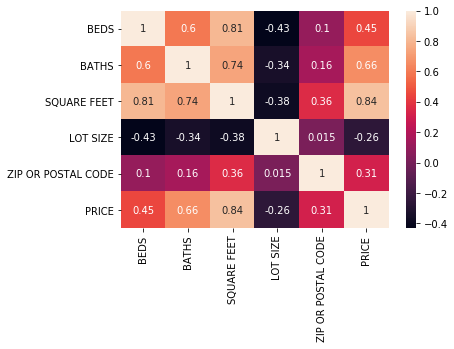### 2.3.1 Explaining the correlation matrix above¶

• Both these tell us that BEDS, BATHS are very strongly correlated to SQUARE FEET
• (BEDS,SQUARE FEET) - very strong correlation
• (BATHS,SQUARE FEET) - very strong correlation
• We can keep one of BEDS,BATHS,SQUARE FEET but (BEDS,BATHS) are not as strong indicators of each other as SQUARE FEET
• (BEDS,BATHS) - strong correlation not that significant
• Looking at the above we can probably discard BEDS and BATHS
• Let's look at (SQUARE FEET,LOT SIZE) the negative correlation makes sense that as SQUARE FEET grows LOT SIZE likely shrinks It is correlated but it's not very strong as in the previous cases.
• (LOT SIZE,SQUARE FEET) - we can probably keep both these features
• ZIP OR POSTAL CODE atcually looks very promising looking at it's correlation matrix - this is a prime candidate for further exploration

### 2.4.0 Dealing with missing values¶

• SQUARE FEET - setting value to 0 - these are just empty land properties
• LOT SIZE - setting value to 0 - these are zero-lot-line houses with structures almost at the end of the property
• ZIP CODE next and it doesn't have any missing data so it seems to be our lucky day here
In :
cols = ['SQUARE FEET','PRICE','LOT SIZE','BEDS','BATHS']
for col in cols:
df[col].fillna(0,inplace=True)


### 2.5.0 Exploring other features¶

• Looking at the data one feature that jumps out is the ZIP CODE
• This however is categorical data
• Since the house listings are nicely grouped into just a few ZIP CODE's, we can use some thing called one-hot encoding
• This basically represents each unique ZIP CODE as a new feature and each row marks 1 IF IT BELONGS 0 IF IT DOES NOT BELONG to the ZIP CODE
• You can read more about one hot encoding to get a clearer understanding here
In :
# one hot encoding the ZIP CODE feature
ohe_df = pd.get_dummies(df['ZIP OR POSTAL CODE'],prefix='ZIP',drop_first=True)
df = pd.concat([df,ohe_df],axis=1)


### 2.5.1 Determining the viability of the new features¶

In :
# covariance matrix of the ohe concat matrix
correlation = df[['PRICE','BEDS','BATHS','SQUARE FEET','ZIP_98012','ZIP_98021','ZIP_98028','ZIP_98036']].corr()
sns.heatmap(correlation,annot=True)
df = df[['PRICE','BEDS','BATHS','SQUARE FEET','ZIP_98012','ZIP_98021','ZIP_98028','ZIP_98036']]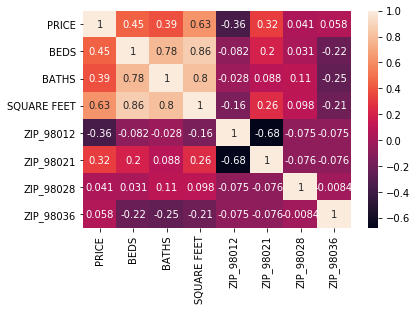### 2.5.2 Explaining the above correlation matrix¶

• The Values above look very promising as they do not have a strong correlation with each other
• What this implies is that each feature that we selected can contribute something new to our regression model

### 2.6.0 Visualizing the data¶

• Looking at the scatter plot for all the different pair wise planes basically tells us that, amongst the numerical features the one's with the lowest amount of spread most likely don't contribute anything significant to our linear regression model
• This gives us a more intuitive feel for the spread of the data
• I've also grouped the feature histograms by postal codes get a sense of their spread over the same neighborhood
In :
# The pairwise plot of each of the features - giving us a sense of the spread of the data
sns.pairplot(df_temp,hue='ZIP OR POSTAL CODE',diag_kind='hist')
plt.show()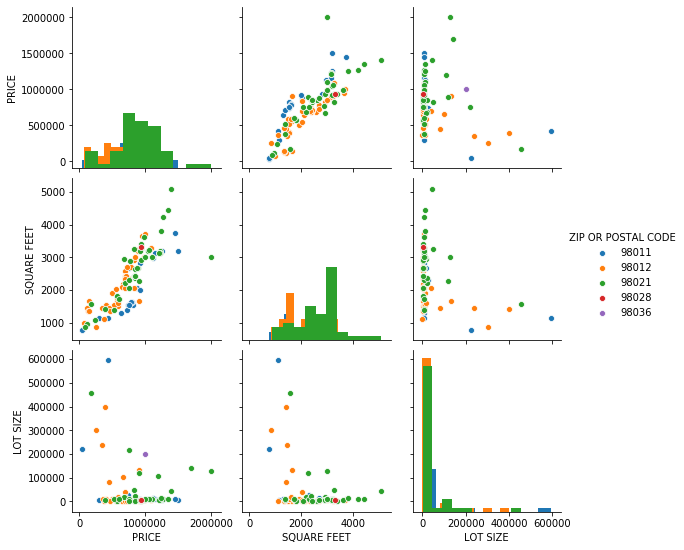### 3.1.0 Splitting data sets¶

• Original Feature space Final Feature space
27 7
• Training Data Validation Data Test Data Total Data Points
60% 20% 20% 120
In :
# doing a randomized split of the dataset so as to reduce training bias

df_train = df.sample(n=72)
df_validation = df.sample(n=24)
df_test = df.sample(n=24)

In :
# Data point counts

print('Training Data : ',df_train.shape)
print('Validation Data : ',df_validation.shape)
print('Test Data : ',df_test.shape)

Training Data :  (72, 8)
Validation Data :  (24, 8)
Test Data :  (24, 8)


### 4.1.0 Linear Regression - Using The Normal Equation¶

• Our Goal is to find weights $\vec{\omega}$ that model the data as closely as possible.
• The model $h(\vec{X})$ is a weighted linear combination of these weights $\omega_i \in \vec{\omega}$ and their respective features $x_i \in \vec{X}$
• $h(\vec{X}) = \vec{\omega}^t \vec{X}$
• $h(x) = \omega_0 + \omega_1x_1 + \omega_2x_2 ... \omega_nx_n$
• $\vec{\omega} = (X^T.X + \epsilon I)^{-1}.X^T.Y$ $I$ is the identity matrix of suitable dimension
In :
# helper functions

def train(df,num_features,epsilon):
column = np.full(df.shape,1.0)
mat_xy = df.to_numpy()
X = mat_xy[:,1:num_features+1]
X = np.insert(X,0,column,axis=1)  #accounting for w0
Y = mat_xy[:,0]
W = np.linalg.inv(X.T.dot(X) + epsilon*np.identity(X.shape)).dot(X.T).dot(Y)
return W

def predict(df,w):
column = np.full(df.shape,1.0)
mat_xy = df.to_numpy()
mat_xy = np.insert(mat_xy,1,column,axis=1)  #accounting for w0
return mat_xy[:,1:(len(w)+1)].dot(w)

def plot(df,feature):
plt.plot(df[feature],df['PRICE'],'.',color='xkcd:azure',label='REAL PRICE')
plt.plot(df[feature],df['PREDICTED PRICE'],'+',color='xkcd:orange',label='PREDICTED PRICE')
plt.xlabel(feature)
plt.ylabel('PRICE')
plt.legend(loc='best')
plt.show()


### 4.2.0 Linear Regression - Model Training¶

In :
W = train(df_train,7,0.00000000000000000000000000000001)


### 4.3.0 Linear Regression - Model Prediction¶

In :
df_validation['PREDICTED PRICE'] = predict(df_validation,W)

Out:
PRICE BEDS BATHS SQUARE FEET ZIP_98012 ZIP_98021 ZIP_98028 ZIP_98036 PREDICTED PRICE
109 994925 5 3.00 3004.0 0 1 0 0 9.328092e+05
74 849990 3 2.50 2376.0 0 1 0 0 9.001544e+05
39 989000 4 2.75 3607.0 0 1 0 0 1.240862e+06
50 1099950 4 2.50 3004.0 0 1 0 0 1.040521e+06
55 1345990 7 3.75 4438.0 0 1 0 0 1.237614e+06

### 4.4.0 Linear Regression - Model Validation against scikit¶

• We've finished training our model and running it on validation data, but if we don't have any standard to compare it with then we basically don't know if the model is working as it should

• Let's use scikit-learn on the same data and check if got the correct values for the weights w

In :
# cross checking my model against scikit-learn's Linear Regressor

regr = linear_model.LinearRegression()
regr.fit(df_train.iloc[:,1:].values,df_train.iloc[:,0].values)

Out:
LinearRegression(copy_X=True, fit_intercept=True, n_jobs=None, normalize=False)
In :
# comparing feature weights

df_weights = pd.DataFrame(W[1:],columns=['MY WEIGHTS'])
df_weights['SCIKIT WEIGHTS'] = pd.DataFrame(regr.coef_,columns=['SCIKIT WEIGHTS'])

Out:
MY WEIGHTS SCIKIT WEIGHTS
0 -81782.129689 -81782.129689
1 -51859.982718 -51859.982718
2 353.740584 353.740584
3 -211314.738416 -211314.738416
4 -75952.548373 -75952.548373

### 4.4.1 Linear Regression - Model Testing¶

In :
df_test['PREDICTED PRICE'] = predict(df_test,W)

Out:
PRICE BEDS BATHS SQUARE FEET ZIP_98012 ZIP_98021 ZIP_98028 ZIP_98036 PREDICTED PRICE
67 299500 2 2.0 1152.0 0 0 0 0 6.508406e+05
114 735000 3 2.0 2300.0 0 0 0 0 9.751526e+05
113 900000 0 0.0 1653.0 1 0 0 0 8.840341e+05
57 918800 4 3.5 2000.0 0 0 0 0 7.094584e+05
73 1221995 4 3.0 3181.0 0 1 0 0 1.077203e+06

### 5.1.0 Model Results¶

• We've finished training, validating and testing out model - let's now actually graphically see our results
• We're gonna plot the numerical features against PRICE and actually compare it with the PREDICTED PRICE that was our prediction
• You can't really draw a line in 2D based off one feature - because the model takes in data points that have all the features and then outputs a price prediction
• However you can see a clear trend amongst the predictions which actually approximates an n-dimensional regression line
• Let's see some colorful graphs now
In :
# plotting features vs price comparision graphs

plot(df_test,'SQUARE FEET')
plot(df_test,'BEDS')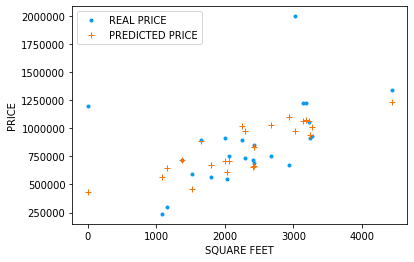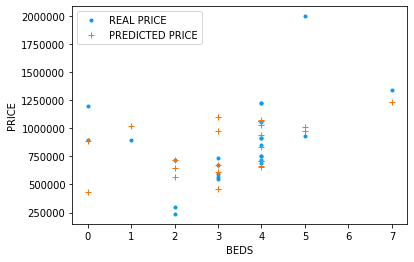### 5.2.0 Model Performance and comparision¶

• We can't really give an accuracy score for this model - because it is a regression based model, and talking about accuracy scores makes sense only in classification problems
• $R_{ss}$ is the sum squared regression error calculated as $R_{ss} = \sum(y_{i} - y_{regression})^2$
• $T_{ss}$ is the sum squared total error calculated as below $T_{ss} = \sum(y_{i} - \overline{y})^2$
• However we can estimate the $R^2$ score of the model which is calculated as below
• $R^2 = 1 - \dfrac{R_{ss}}{T_{ss}}$
In :
df_test.head()

Out:
PRICE BEDS BATHS SQUARE FEET ZIP_98012 ZIP_98021 ZIP_98028 ZIP_98036 PREDICTED PRICE
67 299500 2 2.0 1152.0 0 0 0 0 6.508406e+05
114 735000 3 2.0 2300.0 0 0 0 0 9.751526e+05
113 900000 0 0.0 1653.0 1 0 0 0 8.840341e+05
57 918800 4 3.5 2000.0 0 0 0 0 7.094584e+05
73 1221995 4 3.0 3181.0 0 1 0 0 1.077203e+06
In :
# comparing r2 scores of my model vs scikit learn

my_r2 = r2_score(df_validation.iloc[:,0],regr.predict(df_validation.iloc[:,1:8]))
scikit_r2 = r2_score(df_validation.iloc[:,0],predict(df_validation,W))
df_r2 = pd.DataFrame([[my_r2,scikit_r2]],columns=['MY R2 SCORE','SCIKIT R2 SCORE'])